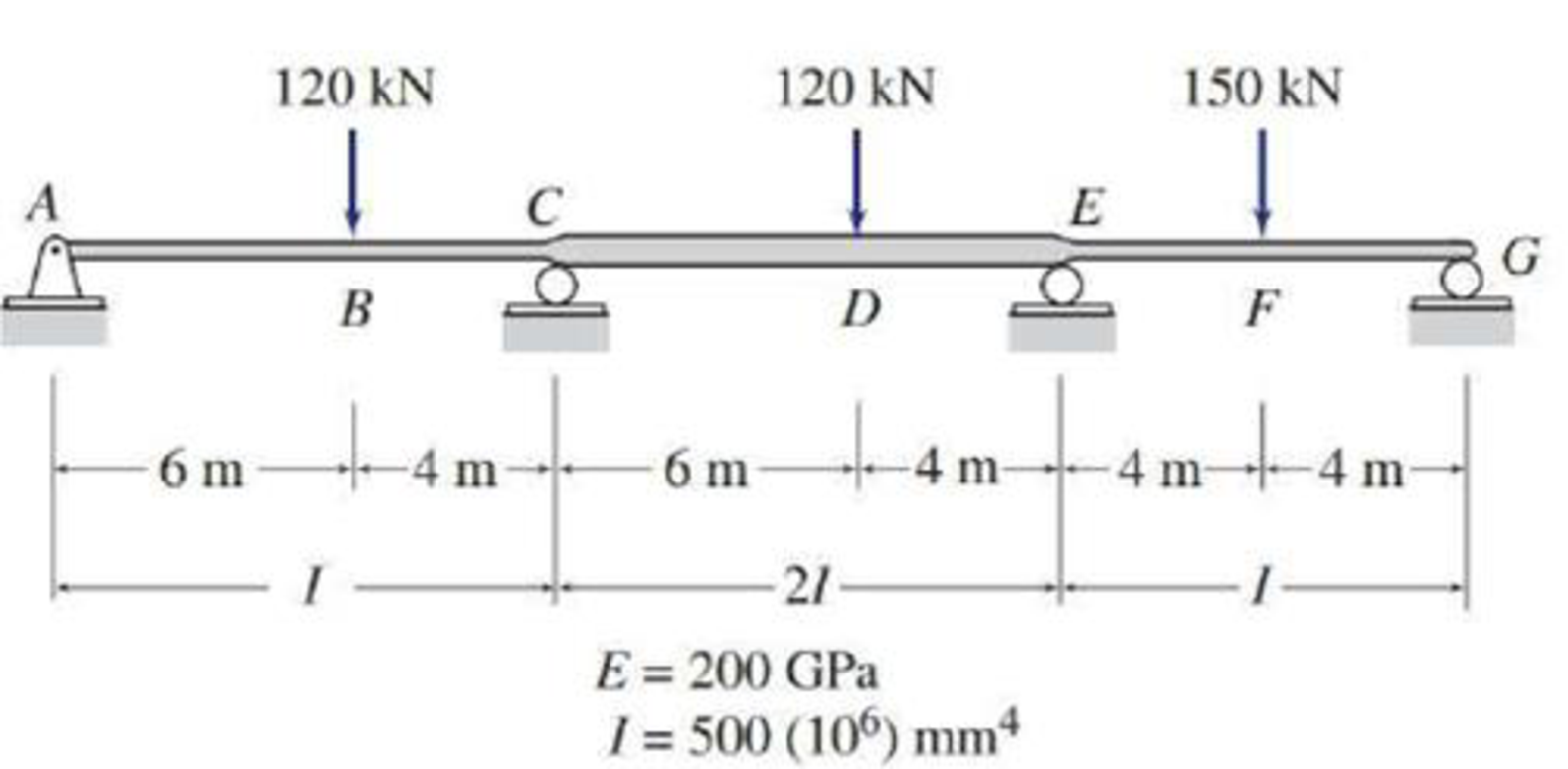# Solve Problem 13.39 for the loading shown in Fig. P13.39 and the support settlements of 10 mm at A , 65 mm at C , 40 mm at E , and 25 mm at G . 13.37 through 13.45 Determine the reactions and draw the shear and bending moment diagrams for the structures shown in Figs. P13.37–P13.45 using the method of consistent deformations. FIG.P13.39, P13.54

#### Solutions

Chapter
Section
Chapter 13, Problem 54P
Textbook Problem
16 views

## Solve Problem 13.39 for the loading shown in Fig. P13.39 and the support settlements of 10 mm at A, 65 mm at C, 40 mm at E, and 25 mm at G.13.37 through 13.45 Determine the reactions and draw the shear and bending moment diagrams for the structures shown in Figs. P13.37–P13.45 using the method of consistent deformations.FIG.P13.39, P13.54

To determine

Calculate the support reactions for the given beam using method of consistent deformation.

Sketch the shear and bending moment diagrams for the given beam.

### Explanation of Solution

Given information:

The settlement at A (ΔA) is 10mm=0.01m.

The settlement at C (ΔC) is 65mm=0.065m.

The settlement at E (ΔE) is 40mm=0.04m.

The settlement at G (ΔG) is 25mm=0.025m.

The moment of inertia (I) is 500×106mm4.

The modulus of elasticity (E) is 200 GPa.

The structure is given in the Figure.

Apply the sign conventions for calculating reactions, forces and moments using the three equations of equilibrium as shown below.

• For summation of forces along x-direction is equal to zero (Fx=0), consider the forces acting towards right side as positive (+) and the forces acting towards left side as negative ().
• For summation of forces along y-direction is equal to zero (Fy=0), consider the upward force as positive (+) and the downward force as negative ().
• For summation of moment about a point is equal to zero (Matapoint=0), consider the clockwise moment as negative and the counter clockwise moment as positive.

Calculation:

Sketch the free body diagram of the structure as shown in Figure 1.

Calculate the degree of indeterminacy of the structure:

Degree of determinacy of the beam is equal to the number of unknown reactions minus the number of equilibrium equations.

The beam is supported by 5 support reactions and the number of equilibrium equations is 3.

Therefore, the degree of indeterminacy of the beam is i=2.

Select the bending moments at the supports E and C as redundant.

Let θCL and θCR are the slopes at the ends C of the left and right spans of the primary beam due to external loading.

Let θEL and θER are the slopes at the ends E of the left and right spans of the primary beam due to external loading.

Let fCCL and fCCR are the flexibility coefficient representing the slopes at C of the left and right spans due to unit value of redundant MC.

Let fEEL and fEER are the flexibility coefficient representing the slopes at E of the left and right spans due to unit value of redundant ME.

Let fCE be the flexibility coefficient representing the slope at point C of the primary beam due to unit value at point E.

Use beam deflection formulas:

Calculate the value of θCL using the relation:

θCL=Pa(L2a2)6EIL

Substitute 120kN for P, 6m for a, and 10m for L.

θCL=120×6(10262)6EI(10)=768kNm2EI

Substitute 500×106mm4 for E and 200 GPa for E.

Calculate the value of θCR using the relation:

θCR=Pb(L2b2)6EIL

Substitute 120kN for P, 4m for b, 2I for I, and 10m for L.

θCR=120×4(10242)6E(2I)(10)=336kNm2EI

Substitute 500×106mm4 for E and 200 GPa for E.

Calculate the value of θEL using the relation:

θEL=Pa(L2a2)6EIL

Substitute 120kN for P, 6m for a, 2I for I, and 10m for L.

θEL=120×6(10262)6E(2I)(10)=384kNm2EI

Substitute 500×106mm4 for E and 200 GPa for E.

Calculate the value of θER using the relation:

θER=PL216EI

Substitute 150kN for P and 8m for L.

θER=150(8)216EI=600kNm2EI

Substitute 500×106mm4 for E and 200 GPa for E.

Calculate the value of fCCL using the relation:

fCCL=ML3EI

Substitute 1kNm for M and 10 m for L.

fCCL=1(10)3EI=10kNm2/kNm3EI

Substitute 500×106mm4 for E and 200 GPa for E.

Calculate the value of fCCR using the relation:

fCCR=ML6EI

Substitute 1kNm for M and 10 m for L.

fCCL=1(10)6EI=10kNm2/kNm6EI

Substitute 500×106mm4 for E and 200 GPa for E.

Calculate the value of fCE and fEC using the relation:

fCE=fEC=ML6EI

Substitute 1kNm for M, 2I for I, and 10 m for L.

fCE=fEC=1(10)6E(2I)=10kNm2/kNm12EI

Substitute 500×106mm4 for E and 200 GPa for E.

Calculate the value of fEEL using the relation:

fEEL=ML6EI

Substitute 1kNm for M and 10 m for L.

fEEL=1(10)6EI=10kNm2/kNm6EI

Substitute 500×106mm4 for E and 200 GPa for E.

fEEL=10kNm2/kNm6×200GPa×106kN/m21GPa×500×106mm4×(1m1,000mm)4=0

### Still sussing out bartleby?

Check out a sample textbook solution.

See a sample solution

#### The Solution to Your Study Problems

Bartleby provides explanations to thousands of textbook problems written by our experts, many with advanced degrees!

Get Started

Find more solutions based on key concepts
Calculate the R-value for the following materials: a. 4 in. thick brick b. 10 cm thick brick c. 12 in. thick co...

Engineering Fundamentals: An Introduction to Engineering (MindTap Course List)

What enterprise resource planning (ERP)? What is supply chain management (SCM)?

Systems Analysis and Design (Shelly Cashman Series) (MindTap Course List)

The _____ is the main circuit board of the computer. (278) a. ALU b. CPU c. motherboard d. system chassis

Enhanced Discovering Computers 2017 (Shelly Cashman Series) (MindTap Course List)

Name and describe the most prevalent characteristics of cloud computing services.

Database Systems: Design, Implementation, & Management

Why does a band saw blade need to be ground after welding?

Precision Machining Technology (MindTap Course List)

Work is calculated by multiplying by.

Automotive Technology: A Systems Approach (MindTap Course List)

What is the name of the eye burn that can occur in a fraction of a second?

Welding: Principles and Applications (MindTap Course List)

If your motherboard supports ECC DDR3 memory, can you substitute non-ECC DDR3 memory?

A+ Guide to Hardware (Standalone Book) (MindTap Course List)